You can define mutually dependent nodes, including a loop decision node named "end", on a do-while node. DataWorks repeatedly runs the nodes and exits the loop only when the end node returns False.

Note A loop can be repeated for a maximum of 128 times. If the loop count exceeds this limit, an error occurs.

The do-while node supports the MaxCompute SQL, SHELL, and Python languages. If you use MaxCompute SQL, you can use a case statement to evaluate whether the specified condition for exiting the loop is met. The following figure shows the sample code for the end node.

## Simple example

This section describes how to use a do-while node to repeat a loop five times and display the loop count each time the loop runs.

1. On the DataStudio page, click Data Analytics in the left-side navigation pane. Move the pointer over the Create icon and choose Control > do-while.
2. In the Create Node dialog box that appears, set the parameters and click Commit.
3. Double-click the created do-while node and define the loop body.

The do-while node consists of the start, sql, and end nodes.

• The start node marks the startup of a loop and does not have any business effect.
• DataWorks provides the sql node as a sample business processing node. You need to replace the sql node with your own business processing node, for example, a SHELL node named "Display loop count." The following figure shows the sample code for the SHELL node.
• The end node marks the end of a loop and determines whether to start the loop again. In this example, it defines the condition for exiting the loop for the do-while node.

The end node only assigns values True and False, indicating whether to start a loop again or exit the loop. The following figure shows the sample code for the end node.

The \${dag.loopTimes} variable is used in both the "Display loop count" node and the end node. It is a reserved variable of the system. It indicates the loop count and increments from 1. The internal nodes of the do-while node can directly reference this variable.

The value of the \${dag.loopTimes} variable is compared with 5 in the code, limiting the total number of times the loop runs. The value is 1 for the first run, 2 for the second run, and so on. When the loop runs for the fifth time, the value is 5. In this case, the conditional statement \${dag.loopTimes}<5 is False, and the do-while node exits the loop.

4. Run the do-while node.

You can configure the scheduling settings for the do-while node as needed and submit it to O&M for running.

• do-while node: The do-while node is displayed as a whole node in O&M. To view the loop details about the do-while node, right-click the node and select View Internal Nodes.
• Internal loop body: This view is divided into three parts.
• The left pane of the view lists the rerun history of the do-while node. A record is generated for each run of the whole do-while instance.
• The middle pane of the view shows a loop record list. Each record corresponds to each run of the do-while node. The running status of the node for each run is also displayed.
• The right pane of the view shows the details about the do-while node each time the loop runs. You can click a record in the loop record list to view the running status of the corresponding instance.
5. Check the running result.

Access the internal loop body. In the loop record list, click the record corresponding to the third run. The loop count is 3 in the run logs.

You can also view the run logs of the end node that are generated when the loop runs for the third time and for fifth time, respectively.

The conditional statement 3<5 is True when the loop runs for the third time, while the conditional statement 5<5 is False when the loop runs for the fifth time. Therefore, the do-while node exits the loop after the fifth run.

Based on the preceding simple example, the do-while node works in the following process:

1. Run from the start node.
2. Run nodes in sequence based on the defined node dependencies.
3. Define the condition for exiting a loop for the end node.
4. Run the conditional statement of the end node after the loop ends for the first time.
5. Record the loop count as 1 and start the loop again if the conditional statement returns True in the run logs of the end node.
6. Exit the loop if the conditional statement returns False in the run logs of the end node.

## Complex example

Besides the preceding simple scenarios, do-while nodes can also be used in complex scenarios where each row of data is processed in sequence by using a loop. Before processing data in such scenarios, make sure that:

• You have deployed a parent node that can export queried data to the do-while node. You can use an assignment node to meet this condition.
• The do-while node can obtain the output of the parent node. You can configure the context and dependencies to meet this condition.
• The internal nodes of the do-while node can reference each row of data. In this example, the existing node context is enhanced and the system variable \${dag.offset} is assigned to help you reference the context of the do-while node.

This section describes how to use the do-while node to respectively display records 0 and 1 in two rows of the tb_dataset table each time the loop runs.

1. On the DataStudio page, click Data Analytics in the left-side navigation pane. Move the pointer over the Create icon and choose Control > do-while.
2. In the Create Node dialog box that appears, set the parameters and click Commit.
3. Double-click the created do-while node and define the loop body.
1. Create a parent node named "Initialize dataset" for the do-while node. The parent node generates a test dataset.
2. Click Schedule in the upper-right corner to configure a dedicated context for the do-while node. Set Parameter Name to input and Value Source to the output of the parent node.
3. Type the code for the business processing node named "Print each data row."
• `\${dag.offset}`: a reserved variable of DataWorks. This variable indicates the offset of the loop count to 1. The offset is 0 for the first run, 1 for the second run, and so on. The offset equals to the loop count minus 1.
• `\${dag.input}`: the context that you configure for the do-while node. As mentioned above, the do-while node is configured with the input parameter, with Value Source set to the output of the parent node named "Initialize dataset."

The internal nodes of the do-while node can directly use \${dag.\${ctxKey}} to reference the context. In this example, \${ctxKey} is set to input. Therefore, you can use \${dag.input} to reference the context.

• `\${dag.input[\${dag.offset}]}`: The node "Initialize dataset" exports a table. DataWorks can obtain a row of data in the table based on the specified offset. The value of \${dag.offset} increments from 0. Therefore, the displayed results are \${dag.input}, \${dag.input}, and so on until all data in the dataset is displayed.
4. Define the condition for exiting the loop for the end node. As shown in the following figure, the values of \${dag.loopTimes} and \${dag.input.length} are compared. If the value of \${dag.loopTimes} is smaller than that of \${dag.input.length}, the end node returns True and the do-while node continues the loop. Otherwise, the end node returns False and the do-while node exits the loop.
Note The system automatically sets the \${dag.input.length} variable to the number of rows in the array specified by the input parameter based on the context configured for the do-while node.
4. Run the nodes and view the running result.
• The node "Initialize dataset" generates data rows 0 and 1.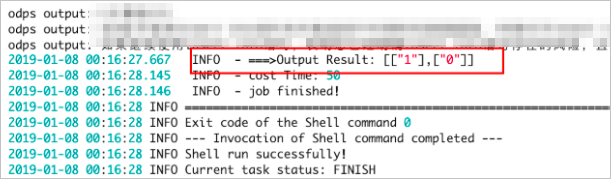• The following figures show the running result of the node "Print each data row."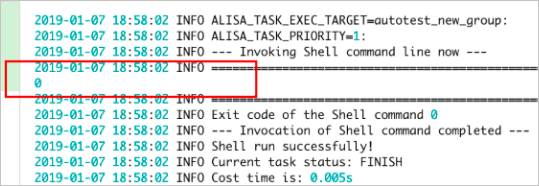Figure 1. Display the first row of data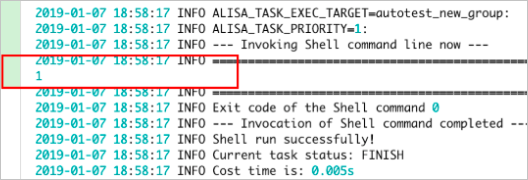Figure 2. Display the second row of data
• The following figures show the running result of the end node.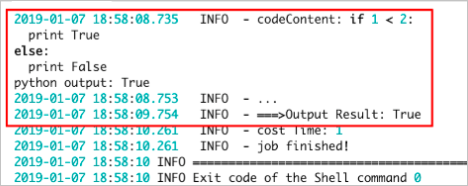Figure 3. Run logs generated when the loop runs for the first time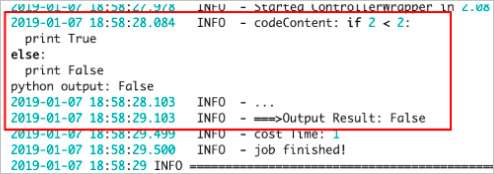Figure 4. Run logs generated when the loop runs for the second time

As shown in the preceding figures, the loop count is smaller than the number of the rows when the loop runs for the first time. Therefore, the end node returns True and the loop continues. The loop count equals to the number of the rows when the loop runs for the second time. Therefore, the end node returns False and the loop stops.

## Summary

• Compared with the while, foreach, and do...while statements, a do-while node:
• Contains a loop body that runs a loop before evaluating the conditional statement, providing the same function as the do...while statement. A do-while node can also use the system variable \${dag.offset} and the node context to implement the function of the foreach statement.
• Cannot achieve the function of the while statement because a do-while node runs a loop before evaluating the conditional statement.
• The do-while node works in the following process:
1. Run nodes in the loop body starting from the start node based on node dependencies.
2. Run the code defined for the end node.
• Run the loop again if the end node returns True.
• Stop the loop if the end node returns False.
• Method to use the context: The internal nodes of the do-while node can use \${dag.\${ctxKey}} to reference the context defined for the do-while node.
• System parameters: DataWorks automatically issues the following system variables for the internal nodes of the do-while node:
• \${dag.loopTimes}: the loop count, starting from 1.
• \${dag.offset}: the offset of the loop count to 1, starting from 0.